# Forecasting with ARIMA¶

Past is a predictor of the future. As businesses grow within reasonable limits (without impact from overly volatile external factors), ARIMA provides a reasonable model-driven predictive capability for risk-averse investing.

ARIMA models are basically sophisticated combination of multiple regression models that extrapolate past behavior to future. This implies an implicit assumption that the factors that behaved a certain way in the past continue to behave relatively similar in the future. This overly simple assumption is one too powerful to keep the models parsimonious, yet effective when compared with deeper econometric regression models. But this simplicity also cedes away the inherent explanability in the model by modeling a complex recipe of contributory factors as a univariate series. But "Keep it Simple" works for most models.

Underneath, we use the number of airline passengers data (between 1949 and 1961) to predict future traffic estimates. Presumably every business has a similar problem; wherein the business must consider past metrics as leading indicators to gauge future demand (and plan capacity).

We use airline passengers data from R data package.

In :
%matplotlib inline
import matplotlib, math
import matplotlib.pyplot as plt
import seaborn as sns
sns.set_context("paper")
sns.set(color_codes=True)

# Get the data and plot
'https://vincentarelbundock.github.io/Rdatasets/csv/datasets/AirPassengers.csv',
usecols=['time', 'AirPassengers'])

# Convert to a date timestamp from the floating point representation of the date
pax_df['time'] = pax_df['time'].apply(lambda x: pd.to_datetime('{0}-{1}-01'.format(str(int(x)), 1 + int(float(format((12.0*(float(x)-int(x))), '.2f')))), format='%Y-%m-%d'))

# Change into a time series
pax_df = pax_df.set_index('time')

# Display a preview of the passenger traffic
pd_display(pax_df, 'Airline passengers by month')


#### Airline passengers by month

AirPassengers
time
1949-01-01 112
1949-02-01 118
1949-03-01 132
... ...
1960-10-01 461
1960-11-01 390
1960-12-01 432

144 rows × 1 columns

## Visualize¶

Let us plot the series to see if the series is "stationary" meaning there is no widening variance or harmonic seasonality in the data.

In :
# Build a chart of the observed values
pax_df.plot()

Out:
<matplotlib.axes._subplots.AxesSubplot at 0x113abdb90>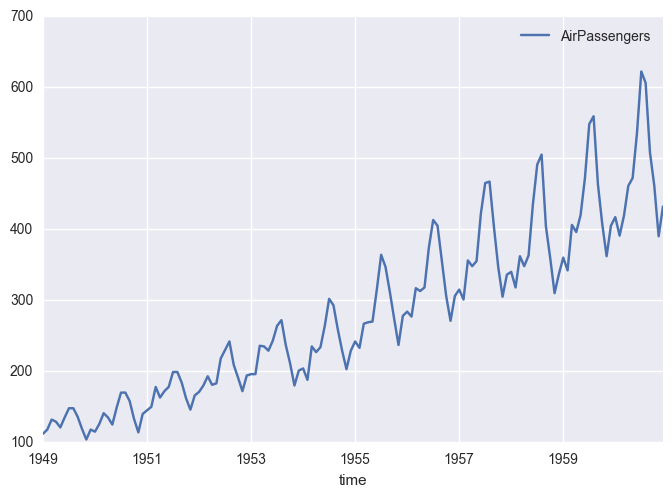## Non Stationarity¶

Just looking at the series visually, we can tell the trend (upward) and the seasonal variance is getting wider (almost exponentially). This suggests that the ARIMA assumption that past factors are stationary are void and violated. We may correct the trend stationarity by differencing (differencing an observation from its prior observation). The seasonal growth (the exponential growth) can be corrected using a logarithmic transformation.

In any case, let us parametrically verify if the said observations (actually the residuals) are stationary.

In :
from statsmodels.tsa.stattools import adfuller

# Turn the data frame into a series
series = pax_df.AirPassengers
series = pd.Series(series, pd.date_range(min(pax_df.index), max(pax_df.index), freq='MS'))

#Perform Dickey-Fuller test:
print 'Results of Dickey-Fuller Test:'
pvaloutput = pd.Series(dftest[0:4], index=['Test Statistic','p-value','#Lags Used','Number of Observations Used'])
print pvaloutput

# Test

Results of Dickey-Fuller Test:
Test Statistic                   0.82
p-value                          0.99
#Lags Used                      13.00
Number of Observations Used    130.00
dtype: float64


The p-value of 0.99 (> 0.05) of the Dickey Fuller Test clearly suggests the series is non-stationary.

To correct it, let us use first differential to remove the upward trend.

In :
#Given a series, do a D=1 first differencing shift
#Window=12 means a seasonal shift (1 year rolling mean shift)
def first_diff(series, numshift):
diff_series = series - series.shift(numshift)
return diff_series.dropna(inplace=False)

# Compute first differencing
first_diff_series = first_diff(series, 1)

# Test if the series has turned stationary

Results of Dickey-Fuller Test:
Test Statistic                  -2.83
p-value                          0.05
#Lags Used                      12.00
Number of Observations Used    130.00
dtype: float64


The p-value of 0.05 suggests that the series may be stationary after computing first difference. Just to visually observe nonetheless, let us plot the first differenced series.

In :
# Plot the first different series
first_diff_series.plot()

Out:
<matplotlib.axes._subplots.AxesSubplot at 0x113b82650>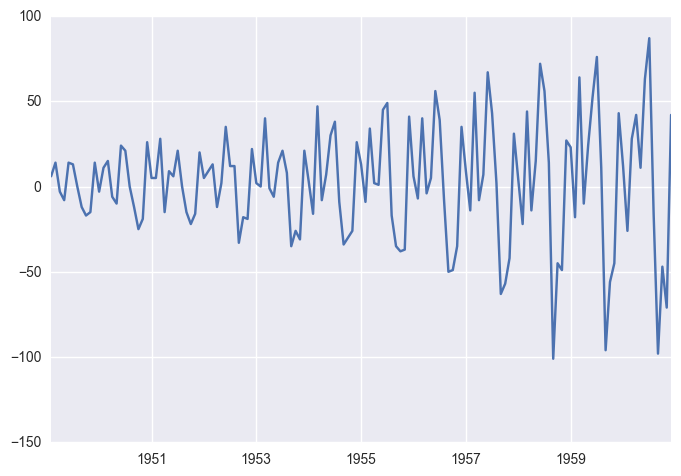While the trend is avoided and the series seems to flutter around the zero mean, the residual seasonal variance is showing a exponential trend. Like stated before, we can correct this by using applying data transformation. Most common transformation is the logarithmic transformation.

In :
# Compute logarithmic transformation
from math import log
log_first_diff_series = lambda nlag: first_diff(series.apply(lambda x: log(x)), nlag)

# Plot the log first diff series, corrected for both trend and variance stationarity
f, ax = plt.subplots(2, sharex=True)
series.apply(lambda x: log(x)).plot(ax=ax)
log_first_diff_series(1).plot(ax=ax)

Out:
<matplotlib.axes._subplots.AxesSubplot at 0x114e9ded0>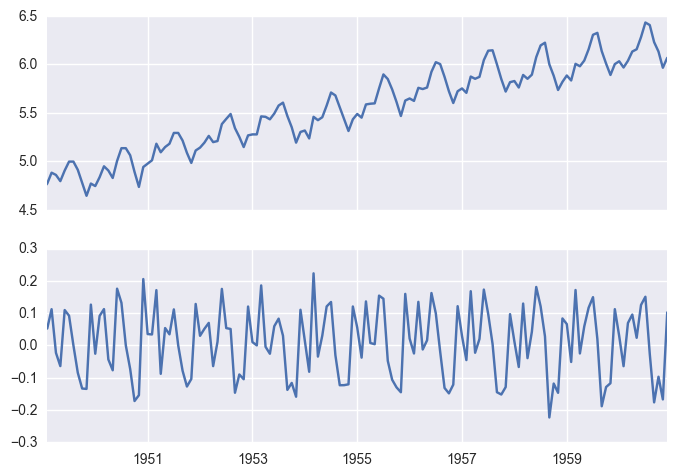## Stationarity¶

The log transformation and the first differencing seems to have helped with the stationarity. Just to ensure, run the Dickey Fuller test again to test.

In :
# Test if the series has turned stationary

Results of Dickey-Fuller Test:
Test Statistic                  -2.72
p-value                          0.07
#Lags Used                      14.00
Number of Observations Used    128.00
dtype: float64


Low p-value suggests that the corrected log first differential (aka D=1) series is near stationary.

In :
# Let us test other lags to see if a better D can be found. At 2, 3, etc

Results of Dickey-Fuller Test:
Test Statistic                  -3.17
p-value                          0.02
#Lags Used                      11.00
Number of Observations Used    130.00
dtype: float64


At D=2, the series is definitely stationary.

## Seasonality, Observed, and Residuals¶

Let us decompose the seasonal, residual, and observed components from the series. We need to do this so we may recompose these components in the forecasted window.

In :
#Given a series of data, let us separate observed trend and seasonal patterns; try to minimize error MSE around zero-mean
def decompose_series(pd_series):
import statsmodels.api as sm
#Decompose the series into monthly segments
decomposition = sm.tsa.seasonal_decompose(pd_series)
#Plot it
fig = decomposition.plot()
fig.set_size_inches(10, 10)

log_series = series.apply(lambda x: log(x))
# Decompose and plot
decompose_series(log_series)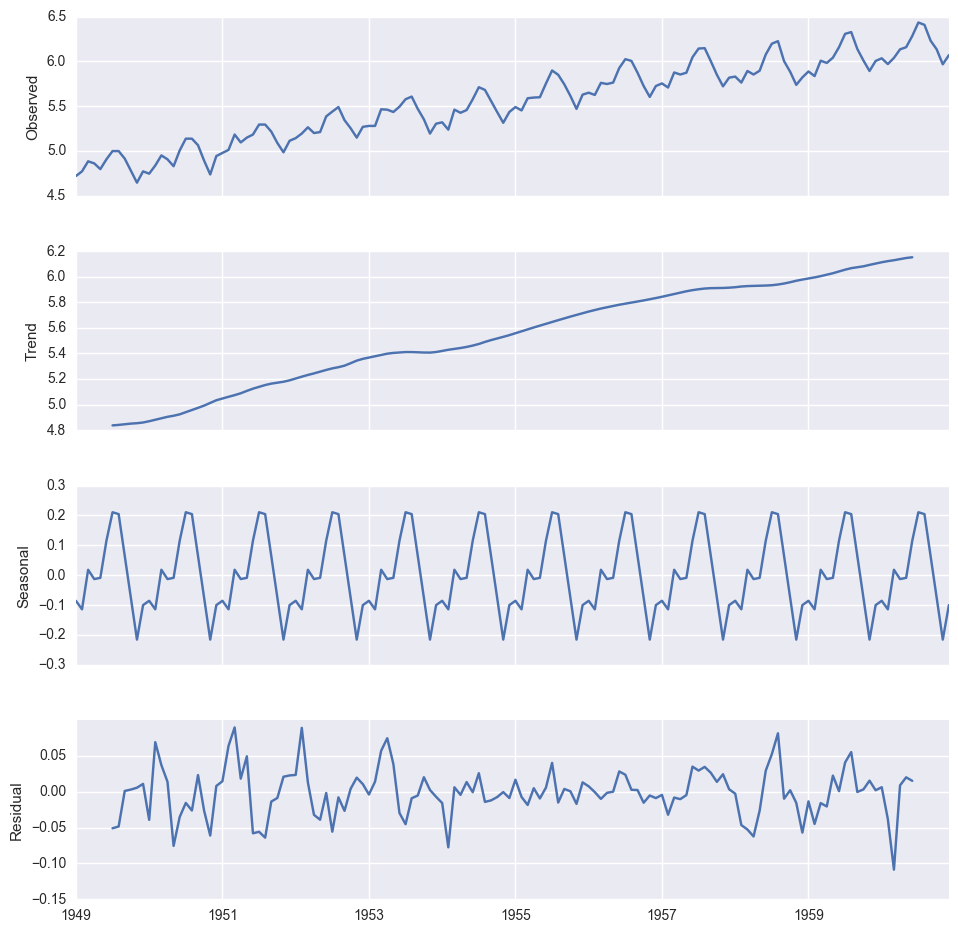The observation shows a definite upward trend in demand. Plus, the travel slumps between fall and the end-of-the-year; it peaks in spring.

## Determining AR/MA Parameters¶

In order to determine the AR and MA parameters, let us look at the PACF and ACF plots respectively.

In :
import statsmodels.api as sm
fig = plt.figure(figsize=(12, 4))
# Raw log plots
fig = sm.graphics.tsa.plot_acf(log_series, lags=24, ax=ax1)
fig = sm.graphics.tsa.plot_pacf(log_series, lags=24, ax=ax2)
# Lag 1 log plots
fig = sm.graphics.tsa.plot_acf(log_first_diff_series(1), lags=24, ax=ax3)
fig = sm.graphics.tsa.plot_pacf(log_first_diff_series(1), lags=24, ax=ax4)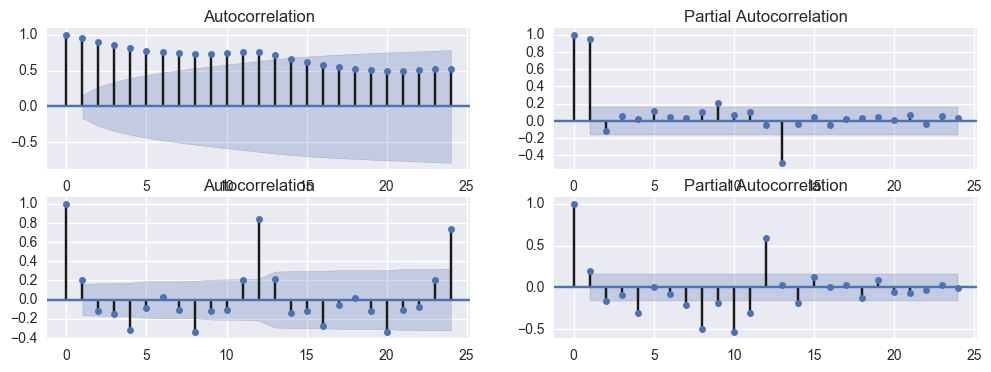The AR and MA parameters of the stationary series (the log first diff series versus the raw series) from PACF and ACF plots suggest 1 and 1 respectively, thus giving rise to (P, D, Q) = (1, 1, 1) respectively.

## Predicting Future¶

To predict the future, let us fit the model

In :
from statsmodels.tsa.statespace.sarimax import SARIMAX
(p, d, q) = (1, 1, 1)

# Fit the model; including seasonality. We have not studied seasonal parameters really, but this will do for the moment.
mod = SARIMAX(log_series, order=(p, d, q), seasonal_order=(p, d, q, 12))
res = mod.fit()

# Create a shell frame for future without any readings first
# Let us project two years out beyond 1960
import datetime
from dateutil.relativedelta import *
date_list = pd.date_range(
start=max(log_series.index), periods=24 + 1, freq='MS')
future = pd.DataFrame(
index=pd.to_datetime(date_list), columns=[log_series.name])
pred_df = pd.concat([log_series.to_frame(), future])

# Predict in the log scale
pred_df['LogPredicted'] = res.predict(start=0, end=len(pred_df.index) + 1)

# Since we are predicting in the log scale, inv log to get real projections
pred_df['ForecastAirPassengers'] = pred_df['LogPredicted'].apply(
lambda x: np.e**x)

# Plot the projections
pd.concat([series, pred_df['ForecastAirPassengers']], axis=1).plot()

Out:
<matplotlib.axes._subplots.AxesSubplot at 0x114c288d0>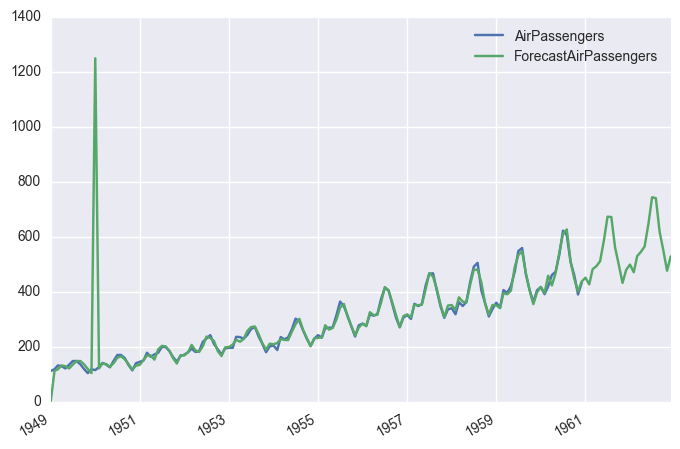## What is the Forecast?¶

In :
# In the next two years what is the likely projected passenger volume?
pax_estimates = pred_df[pred_df.index >= max(series.index)][
'ForecastAirPassengers'].to_frame('Estimated Passengers')
pd_display(pax_estimates, 'Estimated passenger volume for next two years')

# Also plot
pax_estimates.plot(kind='bar')


#### Estimated passenger volume for next two years

Estimated Passengers
1960-12-01 436.63
1960-12-01 436.63
1961-01-01 451.10
... ...
1962-10-01 551.13
1962-11-01 476.31
1962-12-01 528.50

26 rows × 1 columns

Out:
<matplotlib.axes._subplots.AxesSubplot at 0x1157f8290>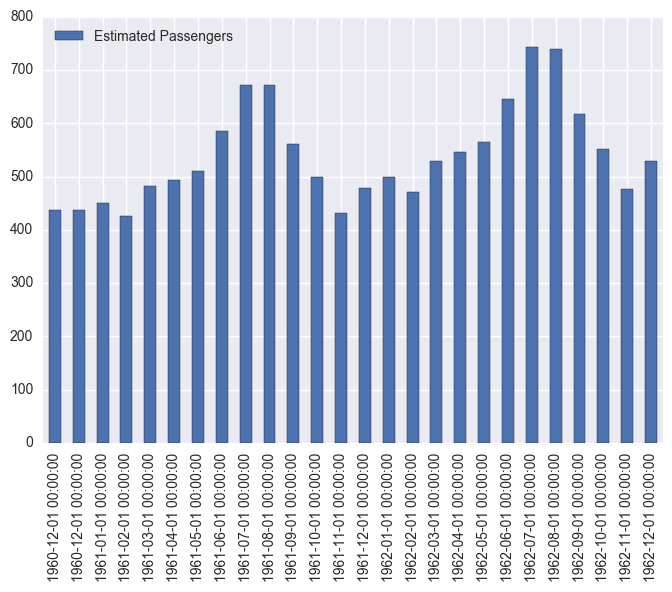## Conclusions¶

BI gives you the descriptive (historic rear-view hindsight) picture. But gaining the predictive prescience and designing preemptive strategies with foresight is very important for every business. To gain some prescience into what the future metrics may look like, simple extrapolative techniques like those offered by ARIMA give good accuracy without getting into overly complex dynamic econometric models. Easily spot seasonality, overall trend, stationarity, and residual components of the past; and recompose a near accurate picture into the future.